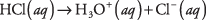## The pH Scale

Even distilled water contains some ions because a small fraction of water molecules dissociates to hydrogen and hydroxide ions:The double arrows indicate that the reaction proceeds either way. This condition of reciprocal reaction is called chemical equilibrium, and its importance to chemistry cannot be overemphasized. An equilibrium state is a stable, balanced condition, and it can be reproduced by many laboratory researchers. It also can be modeled well by simple mathematical equations.

The equilibrium between the water molecule and its ions shows that there are the same number of water molecules forming from union of the two ions as are dissociating into ions. The concentrations of the hydronium and hydroxide ions obey an equilibrium equation:

[H 3O +] [OH ] = K w

where the concentrations are expressed in molarity and K W is the ion‐product constant for water. At a temperature of 25°C, the value of that constant isIn pure water, the concentrations of H 3O + and OH must be equal because the dissociation of H 2O yields the same number of each of them. You can calculate the ionic concentrations from the equilibrium equation and the ion‐product constant:The small value for the ion concentrations shows that in pure water only 1 in every 555,000,000 molecules has dissociated.

The substances classified as acids and bases affect the H 3O + and OH concentrations in solutions, but because the product of the two values must equal K w, increasing one ion must depress the other. An acidic solution has more hydronium ions than hydroxide ions, whereas a basic—or alkaline solution has more hydroxide ions than hydronium ions. (See Table 1.)The use of scientific notation to describe ion concentrations is somewhat cumbersome, and chemists have agreed to employ a pH scale to state the concentration of hydronium ions (the capital “H” in pH stands for hydrogen). They define pH to be the negative logarithm to base 10 of the hydronium ion concentration:

pH = –log 10 [H 3O + ( aq)]

Thus, the pH of neutral water at 25°C is calculated

pH = –log(10 –7) = –(–7) = +7

and the pH of pure water is 7.

Calculate the pH of an acidic solution prepared by adding enough water to 5 grams of hydrochloric acid to make a solution that has a volume of 1 liter.Assuming the hydrochloric acid is completely dissociated,the concentration of the hydronium ions would be 0.137 M, and the pH of the solution equals

pH = –log (0.137) = 0.86

Notice that this acidic pH is a positive number less than 7 because the calculation takes the negative logarithm of [H 3O +]. Try this calculation now to make sure that you understand this point.

The pH of a solution may be estimated from color indicators that change hue with pH, like litmus or phenolphthalein papers; see Table 2. Where precise values are required, an electrical pH meter is utilized.• Calculate the pH of a solution with [OH ] = 2.2 × 10 –6 M, and classify the solution as acidic, neutral, or alkaline.